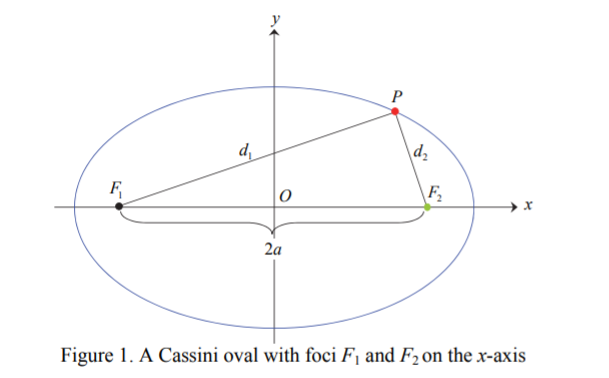# Cassini Oval

A Cassini oval is a plane curve defined as the set of points in the plane with the products of distances to two fixed points (loci) F1 and F2 is constant ; as a formula, the distance is (F1, F2) = 2a .Cassini ovals are generalizations of lemniscates. The ovals are similar to ellipses, but instead of adding distances to loci, you multiply them.

The curve is symmetric with respect to the x-axis, the y-axis, and the origin.

## Equations

The general equation is (x2 + y2 + a2)2 – 4a2x2 = b4.
The polar equation is r4 − 2a2r2cos 2θ + a4.

## Cassini Oval Shapes

The ovals can take on three different shapes, shown in the following image:Cassini ovals. L to R: a = b = 1, a = 0.5 b = 1, a = 1, b = 0.5.

• When a = b, the oval resembles an infinity symbol ∞, which is a Leminiscate of Bernoulli.
• When a < b, the oval takes on the shape of an ellipse or peanut shell.
• If a > b, the oval splits into two ellipses shaped like eggs, with the narrow ends of the “eggs” facing each other. The shapes are mirror-images of each other.

The following image shows the various shapes the Cassini oval can take on, in one image:## Area of Cassini Ovals

The area of a Cassini oval can be found in several ways, including numerical integration and elliptic integrals. What follows is a summary, you can find more detail on pages 235-236 of this PDF.

The oval is symmetric to both the x- and y-axes, so we can find the area of one quarter of an oval, and multiply by 4:You can also calculate the area with the following elliptic integral, where E(x) is the complete elliptic integral of the second kind:## History of the Cassini Oval

Cassini ovals were first studied by 15th Century Giovanni Cassini, who used the ovals to model the sun’s orbit around the Earth . The orbits of the planets around the sun, orbits of satellites around a planet, and electron orbits in atoms, can all be modeled by a Cassini Oval. This may come as a surprise; a common misconception is that planetary orbits are highly elliptical .

Cassini Ovals have a wide variety of uses, including developing radar and sonar systems, modeling human blood cells, and fuel tank optimization.

## References

“Three different shapes” image created with Desmos.
 Mümtaz, K. A Multi Foci Closed Curve: Cassini Oval, Its Properties and Applications. Institutional Archive of the Naval Postgraduate School. 2013. PDF.
 Hellmers, J. et al. Simulation of light scattering by
biconcave Cassini ovals using the nullfield method with discrete sources. Journal of
Optics A: Pure and Applied Optics, Pure Appl. Opt. Vol 8. pp. 1-9. 2006.
 Gibson, K. The ovals of Cassini. Lecture Notes. 2007. Retrieved February 11, 2012 from: https://mse.redwoods.edu/darnold/math50c/CalcProj/sp07/ken/CalcPres.pdf
 Morgado, B. & Soares, V. Kepler’s ellipse, Cassini’s oval and the trajectory of planets. Eur. J. Phys. 35 (2014) 025009.

CITE THIS AS:
Stephanie Glen. "Cassini Oval" From StatisticsHowTo.com: Elementary Statistics for the rest of us! https://www.statisticshowto.com/cassini-oval/